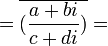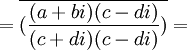## Friday, June 10, 2005

### Norms for Gaussian Integers

In my last blog, I spoke about the importance of unique factorization of integers. This is the idea that all integers can be broken up into a unique set of prime numbers. In today's blog, I will go over some basic lemmas regarding norms. I introduced norms and conjugates in a previous blog.

Carl Friedrich Gauss and Leonhard Euler both proposed extensions of integers that used irrational numbers that they were able to use to make elegant proofs for Fermat's Last Theorem.

A set of integers is extended by including additional values. In standard integers, the values are positive and negative whole numbers and 0. In extended integers, we can include additional values such as i, 5i, etc. Gaussian integers include all of the standard integers plus the addition of multiples of i. In other words, a Gaussian Integer is defined by two integers: a,b where each Gaussian integer can be represented a + bi.

In my previous blog, I spoke about using a special function, the norm, to reason about Gaussian Integers. I will now present a few interesting lemma. To distinguish between Gaussian Integers and standard integers, I will use Greek letters to represent Gaussian Integers and Latin letters to represent standard integers:

Lemma 1: Norm(α) = 0 if and only if α = 0

(1) Norm(α) = Norm(a + bi) = (a + bi)(a - bi) = a2 + b2
(2) But if a ≠ 0, then a2 + b2 > 0.
(3) And the same holds true if b ≠ 0.

QED

In mathematical notation, the conjugate of a Gaussian Integer is represented with an overline over it. So, if α is a Gaussian Integer, then: α is the conjugate. This is important since the norm is equal to a Gaussian Integer multiplied by its conjugate.

Lemma 2: (α * β) = α * β

(1) (α * β) = (a + bi) * (c + di) = (ac - bd) + i(ad + bc)
(2) So, α * β = (ac - bd) - i(ad + bc)
(3) And, α * β = (a - bi) * (c - di) = (ac - bd) - i(ad + bc).
QED

Lemma 3: Norm(α*β) = Norm(α) * Norm(β)

(1) Norm(α*β) = (α*β)(α*β) =
(2) = α*β*α*β [ From Lemma 2 ]
(3) = (α*α)(β*β)
(4) =Norm(α)*Norm(β)

QED

In standard integers, we speak about 1, -1 as being units -- that is, numbers which divide all other numbers. This is important because when we are discussing prime numbers or relatively prime numbers, we are necessarily talking about numbers other than 0 or the units.

In the realm of Gaussian Integers, a unit is defined as any number that divides 1.

Lemma 4: if α is a unit, then Norm(α) = ±1

Proof:

(1) If α is a unit, then there exists another value β such that α * β = 1.
(2) Now, Norm(α * β) = Norm(1) = 1 = Norm(α) * Norm(β)
(3) But if either Norm(α) ≠ ±1 or Norm(β) ≠ ± 1, then the Norm(α * β) couldn't equal 1 (since there are no other rational integers that multiply to 1).
(4) So, Norm(α) must equal ± 1.

QED

Corollary 4.1: The only units for Gaussian Integers are i,-i,1,-1

Proof:

(1) N(i) = i*(-i) = 1 [See Definition 2 here for details if needed]

(2) N(-i) = (-i)*i = 1 [See Definition 2 here for details if needed]

(3) N(1) = 1*1 = 1 [See Definition 2 here for details if needed]

(4) N(-1) = (-1)*(-1) = 1 [See Definition 2 here for details if needed]

(5) If N(α) is a unit where α = a + bi, then:]

(a + bi)(a - bi) = a2 + b2 = 1

(6) But this can only occur if a=0 or b=0 since:

(a) if a ≠ 0 and b≠ 0

(b) Then abs(a) ≥ 1 and abs(b) ≥ 1

(c) And, a2 + b2 ≥ 2

(7) But then the only possible units are 1, -1, i, -i

(a) if a = 0, then b = ± 1 so that we have α = i or α = -i

(b) if b=0, then a = ± 1 so that we have α=1 or α=-1

QED

We are now ready to define a Gaussian Prime. A Gaussian prime is any Gaussian Integer which is not 0 or a unit which is only divisible by a itself or a unit.

Lemma 5: if Norm(α) is a standard prime, then α is a prime.

(1) So, we start with Norm(α) = p which is a standard prime.
(2) Assume α is not a prime.
(3) Then there exists two Gaussian Integers: β, γ that are not units such that: α = β * γ.
(4) And then Norm(β * γ) = Norm(β) * Norm(γ) [By Lemma 3 above]
(5) Since Norm is a mapping function to standard integers, we know that there exists b,g such that:
b = Norm(β)
g = Norm(γ)

(6) We know that Norm(β * γ) = p and we know that b,q ≠ 1 [By Lemma 4, since they are not units]
(7) But then p = b * g which is impossible since p is a prime.
(8) Therefore, we reject our assumption in (2).

QED

Lemma 6: (α/β) = α / β

(1) There exists a,b,c,d such that α = a + bi. β = c + di.

(2) (α/β) =[From Lemma 2 above]= (a + bi) / (c + di)

QED

Lemma 7: Norm(α / β) = Norm(α) / Norm(β)

(1) Norm(α / β) = (α / β) * (α / β) [Definition of Norm]

= (α / β) * α / β = Norm(α) / Norm (β)

QED

Corollary 7.1: If α divides β, then Norm(α) divides Norm(β)

Proof:

(1) Assume that α divides β.

(2) Then there exists an integer γ such that γ = β / α.

(3) By the definition of a norm (see here), Norm(γ) is a rational integer.

(4) But then Norm(β)/Norm(α) = Norm(γ) which means that Norm(α) must divide Norm(β).

QED

In my next blog, I will prove that Gaussian Integers possess unique factorization.

The lemmas from today's blog were taken from an excellent book by Harold M. Stark called An Introduction to Number Theory .

Scouse Rob said...

In Lemma 4 (3) should it be:

"But if either Norm(α) ≠ ±1 ..."

"But if either Norm(α) ≠ 1 ..."

There seems to be no step limiting ±1 to 1 after that.
(I suppose Norm(α)>=0 but this isn't explicitly stated, refer to Lemma 1 (2) perhaps. Maybe it would be better to get rid of the ± stuff before step (3).)

Love all this Gaussian Integer stuff. Fantastic Blog. Rob.

Scouse Rob said...

Eeek!
Those divisions in Lemma 6 (2) were hard to make out. Especially where it was all under one line, it is confusing visually as to whether (a + bi) should be in the denominator or not.

It might be better if you made it:

(a + bi) * (c + di) * 1/[(c + di)(c - di)]

1/[(c + di)(c - di)] * (a + bi) * (c + di)

Rob

Larry Freeman said...

Hi Rob,

Thanks for your comments. Lemma 4 was a typo. I've fixed Lemma 4.

For Lemma 6, I've converted the fractions into images. This should make the divisions much easier to follow.

Cheers,

-Larry